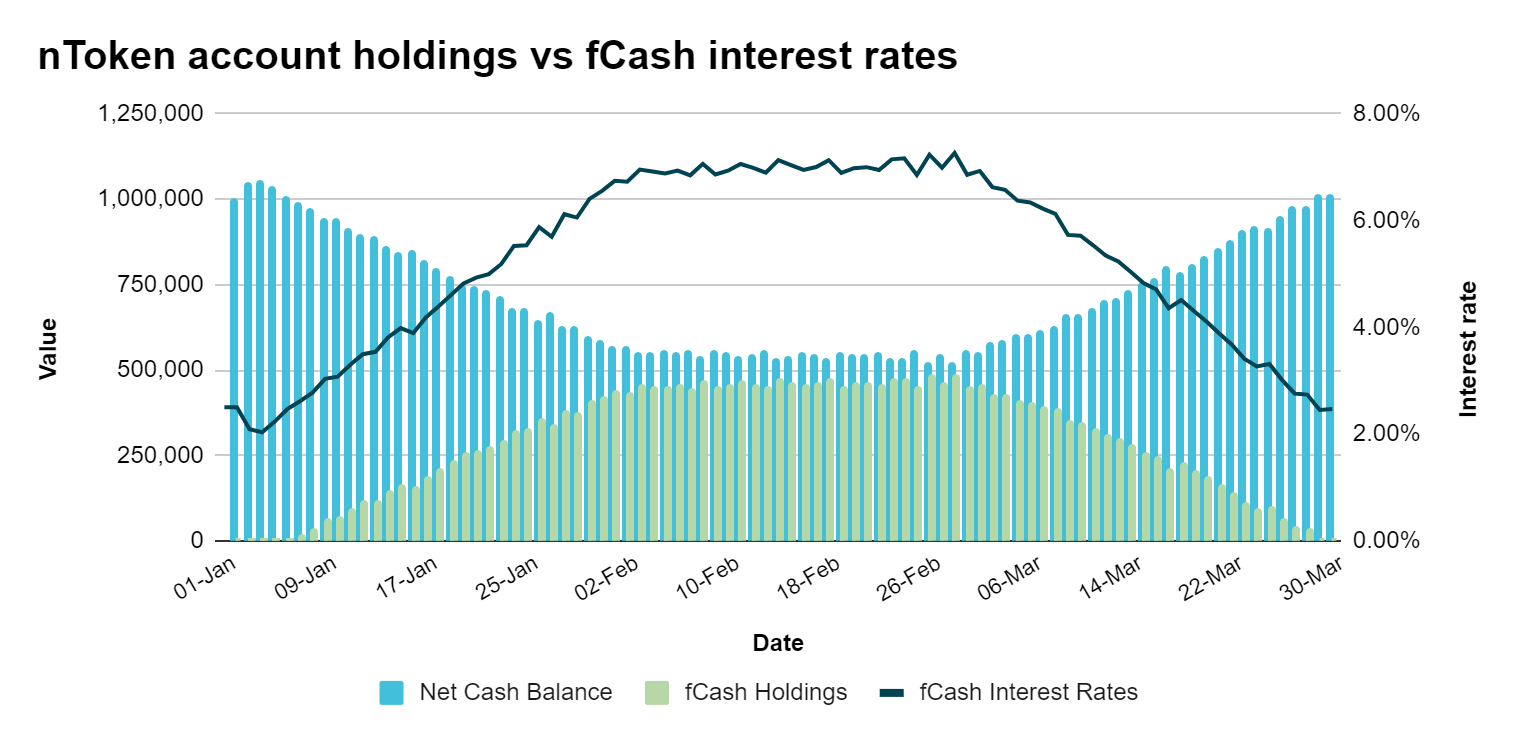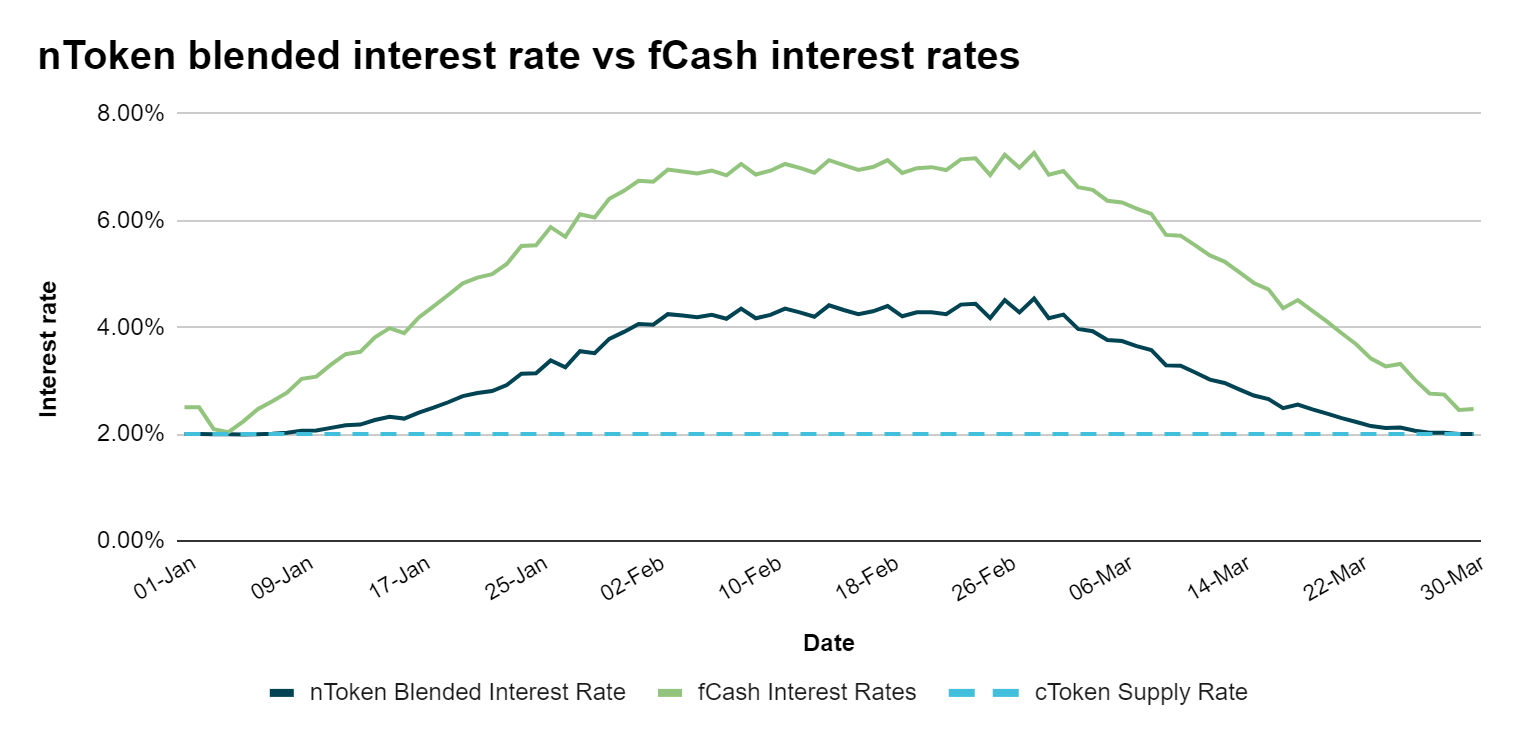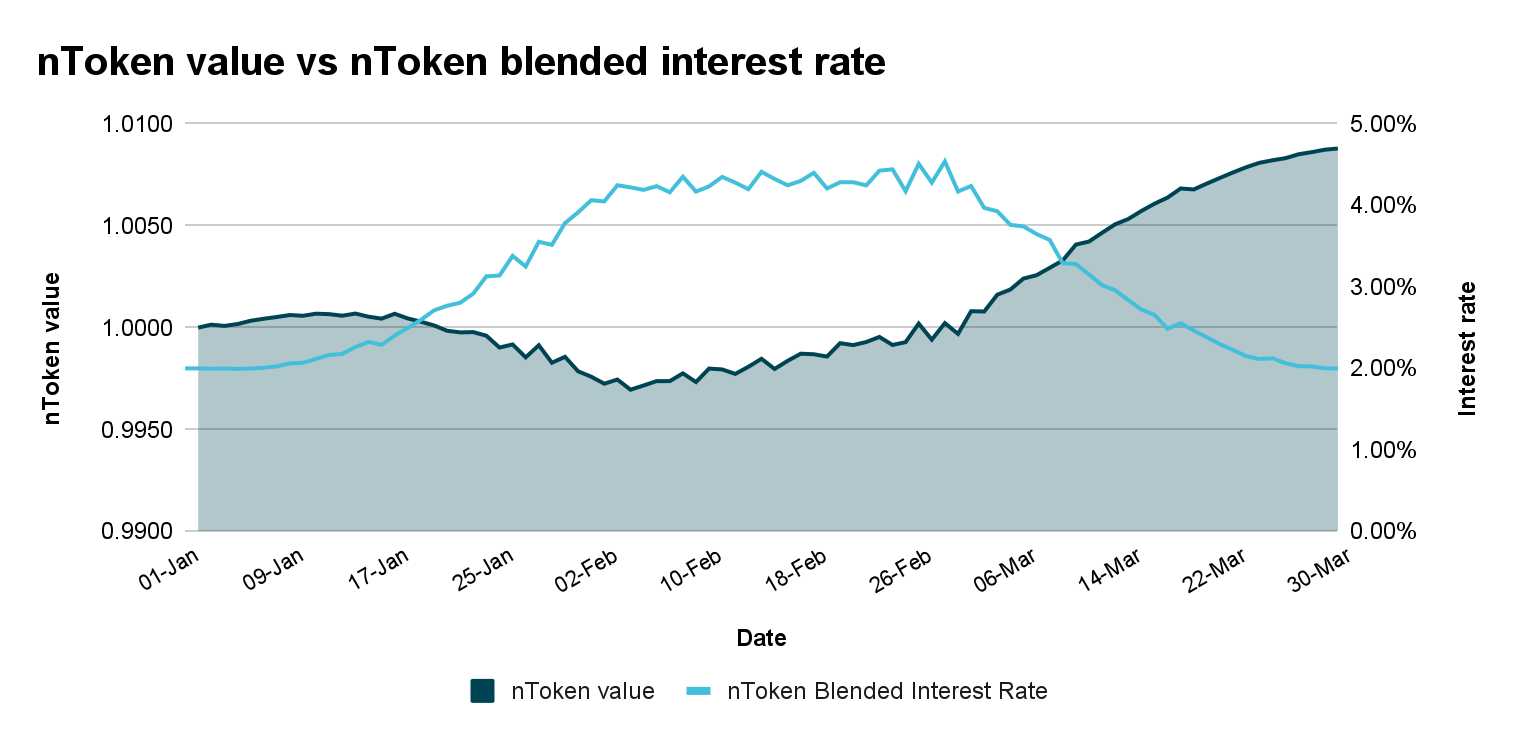# nToken Returns

## Return Drivers

nTokens are a cross between simple interest-bearing assets like cTokens and assets like Uniswap LP tokens. This means that nToken returns are more volatile than cToken returns, but nToken holders are generally rewarded with higher interest rates over time.
nTokens earn returns in three ways:
• Blended interest rate: Interest earned from cTokens and fCash held by the nToken account. The blended interest rate is the average of the cToken interest rate and the fCash interest rates at different maturities weighted by the nToken accounts holdings of cTokens and fCash at each maturity. Find an example calculation of the nToken's blended interest rate here.
• Liquidity Fees / Impermanent Loss: Fees earned from the liquidity tokens held in the nToken account any time an end user borrows or lends. The nToken’s potential for impermanent loss is small because fCash exchange rates are very stable - for example, three month fCash can only trade between an exchange rate of 1 and 1.04.
• NOTE Incentives: nToken holders are directly incentivized with Notional’s governance token, the NOTE. nToken holders accrue NOTE rewards proportional to their share of the total nTokens in that currency.

## Return Dynamics

Here we'll walk through an example scenario that demonstrates these return drivers in action. As interest rates move we'll see how the nToken account's asset holdings change, how the blended interest rate changes, and what impact this has on the nToken's returns.
As end users lend and borrow on Notional’s liquidity pools, they move Notional’s interest rates. This chart shows how changes in Notional's interest rates coincide with changes in the nToken's fCash holdings.When end users borrow and interest rates rise, the nToken account gains fCash and loses cTokens. When end users lend and interest rates fall, the nToken account loses fCash and gains cTokens.
Changes in fCash interest rates also affect the nToken's blended intererst rate. In general, the nToken’s blended interest rate will sit somewhere between the cToken supply rate and the fCash interest rates on Notional. Here is the same example scenario, with the addition of the nToken’s blended interest rate and assuming a constant cToken supply rate of 2%:As the fCash interest rate rises, so does the nToken’s blended interest rate. As the fCash interest rate falls, so does the blended interest rate.
The value of the nToken increases continuously at the blended interest rate but also experiences impermanent loss as interest rates move. This graph of the nToken value against the blended interest rate illustrates how the value of the nToken changes in this scenario:• January: interest rates rose and the nToken experienced impermanent loss that was greater than the blended interest rate earnings. This caused the nToken to decrease in value.
• February: interest rates were stable and the nToken accrued interest at a rate of ~4.5% without experiencing any impermanent loss.
• March: interest rates went back down and the nToken recouped its earlier losses and also continued to accrue interest. This caused the nToken’s value to steeply increase.
The nToken’s annualized return over this period was roughly 4% vs. the assumed cToken supply rate of 2%. nToken returns are more volatile than cToken returns, but nToken holders are rewarded with the liquidity fees and higher interest rates that the nToken accrues over time.